Courses

# Test: Kirchhoff’s Current And Voltage Law

## 20 Questions MCQ Test Networking Theory | Test: Kirchhoff’s Current And Voltage Law

Description
This mock test of Test: Kirchhoff’s Current And Voltage Law for Electrical Engineering (EE) helps you for every Electrical Engineering (EE) entrance exam. This contains 20 Multiple Choice Questions for Electrical Engineering (EE) Test: Kirchhoff’s Current And Voltage Law (mcq) to study with solutions a complete question bank. The solved questions answers in this Test: Kirchhoff’s Current And Voltage Law quiz give you a good mix of easy questions and tough questions. Electrical Engineering (EE) students definitely take this Test: Kirchhoff’s Current And Voltage Law exercise for a better result in the exam. You can find other Test: Kirchhoff’s Current And Voltage Law extra questions, long questions & short questions for Electrical Engineering (EE) on EduRev as well by searching above.
QUESTION: 1

### Find the value of v if v1=20V. Find the value of v if v1=20V and value of current source is 6A.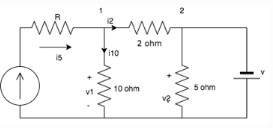Solution:

The current through the 10 ohm resistor=v1/10=2A.Applying KCL at node 1: i5=i10+i2. i2=6-2=4A.
Thus the drop in the 2 ohm resistor= 4×2=8V.
v1=20V; hence v2=20-v across 2 ohm resistor=20-8=12V
v2=v since they are connected in parallel.
v=12V.

QUESTION: 2

### Calculate the current A.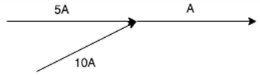Solution:

KCl states that the total current leaving the junction is equal to the current entering it. In this case, the current entering the junction is 5A+10A=15A.

QUESTION: 3

### Calculate the current across the 20 ohm resistor.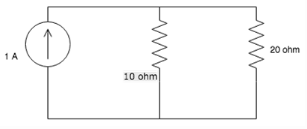Solution:

Assume lower terminal of 20 ohm at 0V and upper terminal at V volt and applying KCL, we get V/10 +V/20 =1. V=20/3V So current through 20 ohm = V/20 = (20/3)/20 =1/3=0.33V.

QUESTION: 4

Calculate the value of I3, if I1= 2A and I2=3A.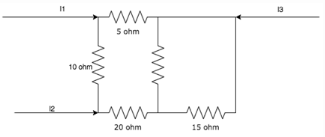Solution:

According to KCl, I1+I2+I3=0. Hence I3=-(I1+I2)=-5A.

QUESTION: 5

Find the value of i2, i4 and i5 if i1=3A, i3=1A and i6=1A.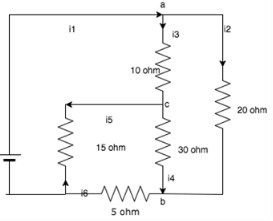Solution:

At junction a: i1-i3-i2=0. i2=2A.
At junction b: i4+i2-i6=0. i4=-1A.
At junction c: i3-i5+i4=0. i5=2A.

QUESTION: 6

What is the value of current if a 50C charge flows in a conductor over a period of 5 seconds?

Solution:

Current=Charge/Time. Here charge = 50c and time = 5seconds, so current = 50/5 = 10A.

QUESTION: 7

KCL deals with the conservation of?

Solution:

KCL states that the amount of charge entering a junction is equal to the amount of charge leaving it, hence it is the conservation of charge.

QUESTION: 8

KCL is applied at _________

Solution:

KCL states that the amount of charge leaving a node is equal to the amount of charge entering it, hence it is applied at nodes.

QUESTION: 9

KCL can be applied for __________

Solution:

KCL is applied for different nodes of a network whether it is planar or non-planar.

QUESTION: 10

What is the value of the current I?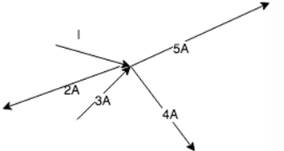Solution:

At the junction, I-2+3-4-5=0. Hence I=8A.

QUESTION: 11

Calculate the value of V1 and V2.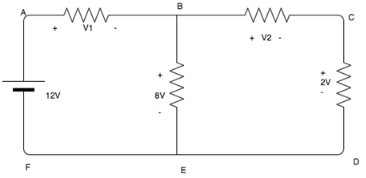Solution:

Using KVL, 12-V1-8=0. V1= 4V.
8-V2-2=0. V2=6V.

QUESTION: 12

KCL deals with the conservation of?

Solution:

KCL states that the amount of charge entering a junction is equal to the amount of charge leaving it, hence it is the conservation of charge.

QUESTION: 13

Calculate the voltage across the 10 ohm resistor.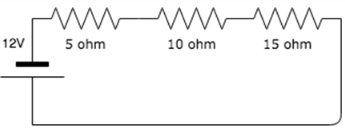Solution:

Using voltage divider rule, V=10*12/30=4V.

QUESTION: 14

Find the value of the currents I1 and I2.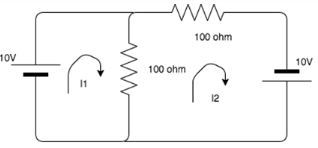Solution:

Using KVL, the matrix to find the loop currents are:
MATRIX 10 -100*i1 + 100*i2= 0
MATRIX  10-200*i2 + 100i1 = 0
I1= 0.3, I2=0.2

QUESTION: 15

The sum of the voltages over any closed loop is equal to __________

Solution:

According to KVL, the sum of the voltage over any closed loop is equal to 0.

QUESTION: 16

What is the basic law that has to be followed in order to analyze ant circuit?

Solution:

Kirchhoff’s laws, namely Kirchhoff’s Current Law and Kirchhoff’s Voltage law are the basic laws in order to analyze a circuit.

QUESTION: 17

Every____________ is a ____________ but every __________ is not a __________

Solution:

According to Kirchhoff’s Voltage Law, Every mesh is a loop but every loop is not a mesh.

QUESTION: 18

Solve for I.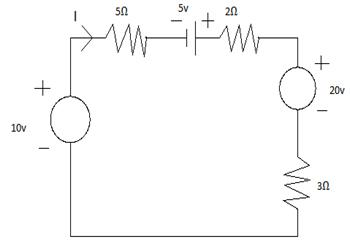Solution:

Veq = 10 + 5 -20 = -5u
Req = 5 + 2 + 3 = 10Ω
I = V/R = -5/10 = -0.5A.

QUESTION: 19

Calculate VAB.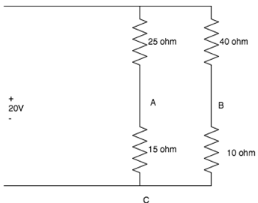Solution:

For branch A: VAC=15*20/(25+15)=7.5V
For branch B: VBC= 10*20/(10+40)=4V
Applying KVL to loop ABC:
VAB+VBC+VCA=0
VAB=3.5V.

QUESTION: 20

KVL is applied in ____________

Solution:

Mesh analysis helps us to utilize the different voltages in the circuit as well as the IR products in the circuit which is nothing but KVL.